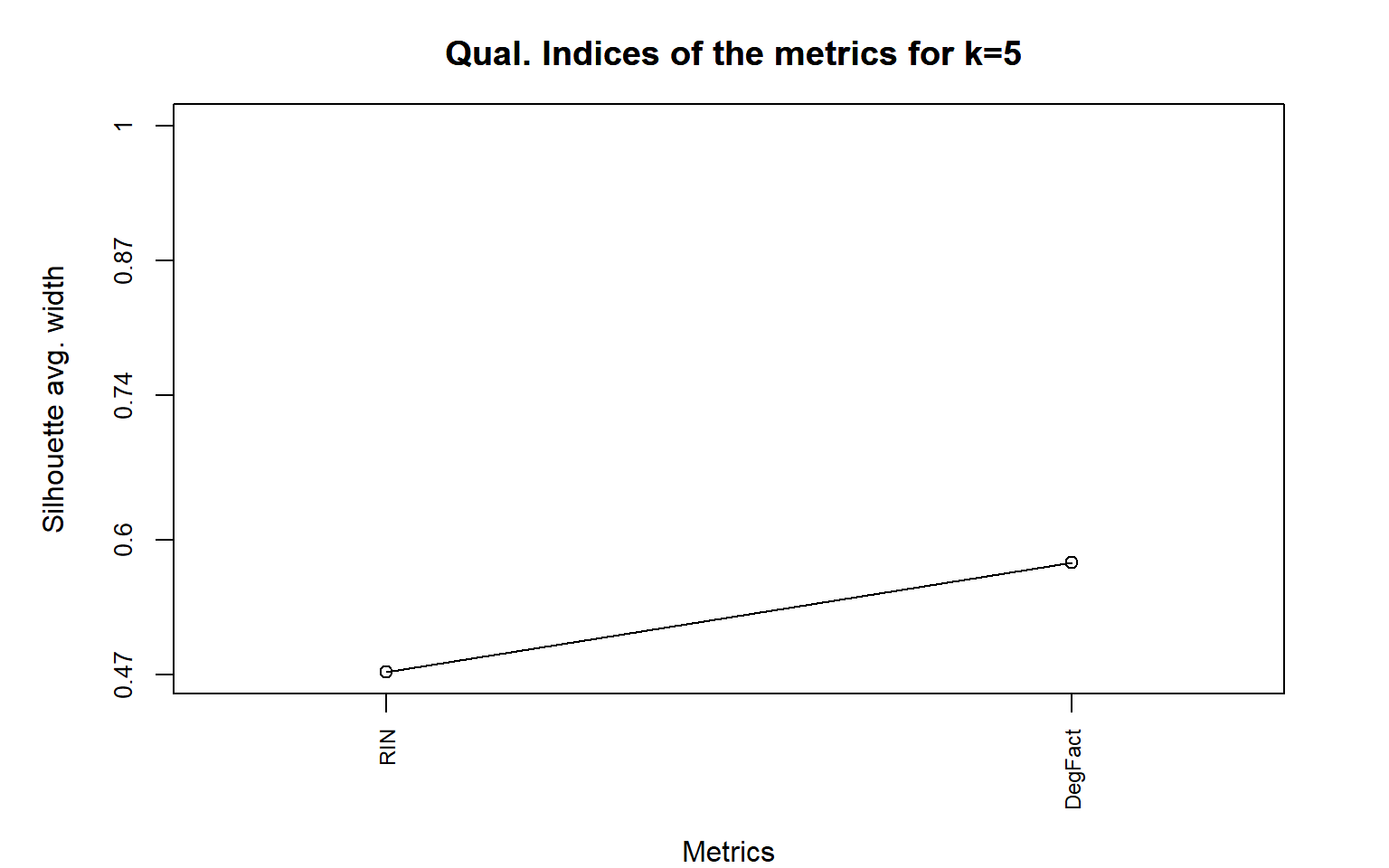# 1 Introduction

The evaluomeR package permits to evaluate the reliability of metrics by analysing the stability and goodness of the classifications of such metrics. The method takes the measurements of the metrics for the dataset and evaluates the reliability of the metrics according to the following analyses: Correlations, Stability and Goodness of classifications.

• Correlations: Calculation of Pearson correlation coefficient between every pair of metrics available in order to quantify their interrelationship degree. The score is in the range [-1,1].

• Perfect correlations: -1 (inverse), and 1 (direct).
• Stability: This analysis permits to estimate whether the clustering is meaningfully affected by small variations in the sample (Milligan and Cheng 1996). First, a clustering using the k-means algorithm is carried out. The value of K can be provided by the user. Then, the stability index is the mean of the Jaccard coefficient (Jaccard 1901) values of a number of bootstrap replicates. The values are in the range [0,1], having the following meaning:

• Unstable: [0, 0.60[.
• Doubtful: [0.60, 0.75].
• Stable: ]0.75, 0.85].
• Highly Stable: ]0.85, 1].
• Goodness of classifications: The goodness of the classifications are assessed by validating the clusters generated. For this purpose, we use the Silhouette width as validity index. This index computes and compares the quality of the clustering outputs found by the different metrics, thus enabling to measure the goodness of the classification for both instances and metrics. More precisely, this goodness measurement provides an assessment of how similar an instance is to other instances from the same cluster and dissimilar to the rest of clusters. The average on all the instances quantifies how the instances appropriately are clustered. Kaufman and Rousseeuw (Kaufman and Rousseeuw 2009) suggested the interpretation of the global Silhouette width score as the effectiveness of the clustering structure. The values are in the range [0,1], having the following meaning:

• There is no substantial clustering structure: [-1, 0.25].
• The clustering structure is weak and could be artificial: ]0.25, 0.50].
• There is a reasonable clustering structure: ]0.50, 0.70].
• A strong clustering structure has been found: ]0.70, 1].

# 2 Installation

The installation of evaluomeR package is performed via Bioconductor:

if (!requireNamespace("BiocManager", quietly=TRUE))
install.packages("BiocManager")
BiocManager::install("evaluomeR")

## 2.1 Prerequisites

The package evaluomeR depends on the following CRAN packages for the calculus: fpc (Hennig 2018), cluster (Maechler et al. 2018), corrplot (Wei and Simko 2017). Moreover, this package also depends on grDevices, graphics, stats and utils from R Core (R Core Team 2018) for plotting and on the Bioconductor package SummarizedExperiment (Morgan et al. 2018) for input/output data.

# 3 Using evaluomeR

## 3.1 Creating an input SummarizedExperiment

The input is a SummarizedExperiment object. The assay contained in SummarizedExperiment must follow a certain structure, see Table 1: A valid header must be specified. The first column of the header is the ID or name of the instance of the dataset (e.g., ontology, pathway, etc.) on which the metrics are measured. The other columns of the header contains the names of the metrics. The rows contains the measurements of the metrics for each instance in the dataset.

Table 1: Example of an input assay from a SummarizedExperiment for the evaluomeR package
ID MetricNameA MetricNameB MetricNameC
instance1 1.2 6.4 0.5
instance2 2.4 5.4 0.8
instance3 1.9 8.9 1.1

## 3.2 Using input sample data from evaluomeR

In our package we provide three different sample input data:

• ont-metrics: Structural ontology metrics, 19 metrics measuring structural aspects of bio-ontologies have been analysed on two different corpora of ontologies: OBO Foundry and AgroPortal (Franco et al. 2019).

• rna-metrics: RNA quality metrics for the assessment of gene expression differences, 2 quality metrics from 16 aliquots of a unique batch of RNA Samples. The metrics are Degradation Factor (DegFact) and RNA Integrity Number (RIN) (Imbeaud et al. 2005).

• biopathways-metrics: Metrics for biological pathways, 2 metrics that quantitative characterizations of the importance of regulation in biochemical pathway systems, including systems designed for applications in synthetic biology or metabolic engineering. The metrics are reachability and efficiency (Davis and Voit 2018).

The user shall run the loadSample method to load evaluomeR sample input data. This requires to provide the descriptor of the desired dataset. The descriptor can take the following values: “ont-metrics”, “rna-metrics” or “biopathways-metrics”.

library(evaluomeR)
biopathwaysMetrics <- loadSample("biopathways-metrics")

## 3.3 Correlations

We provide the correlations function to evaluate the correlations among the metrics defined in the SummarizedExperiment:

library(evaluomeR)
correlationSE <- correlations(rnaMetrics, margins =  c(4,4,12,10))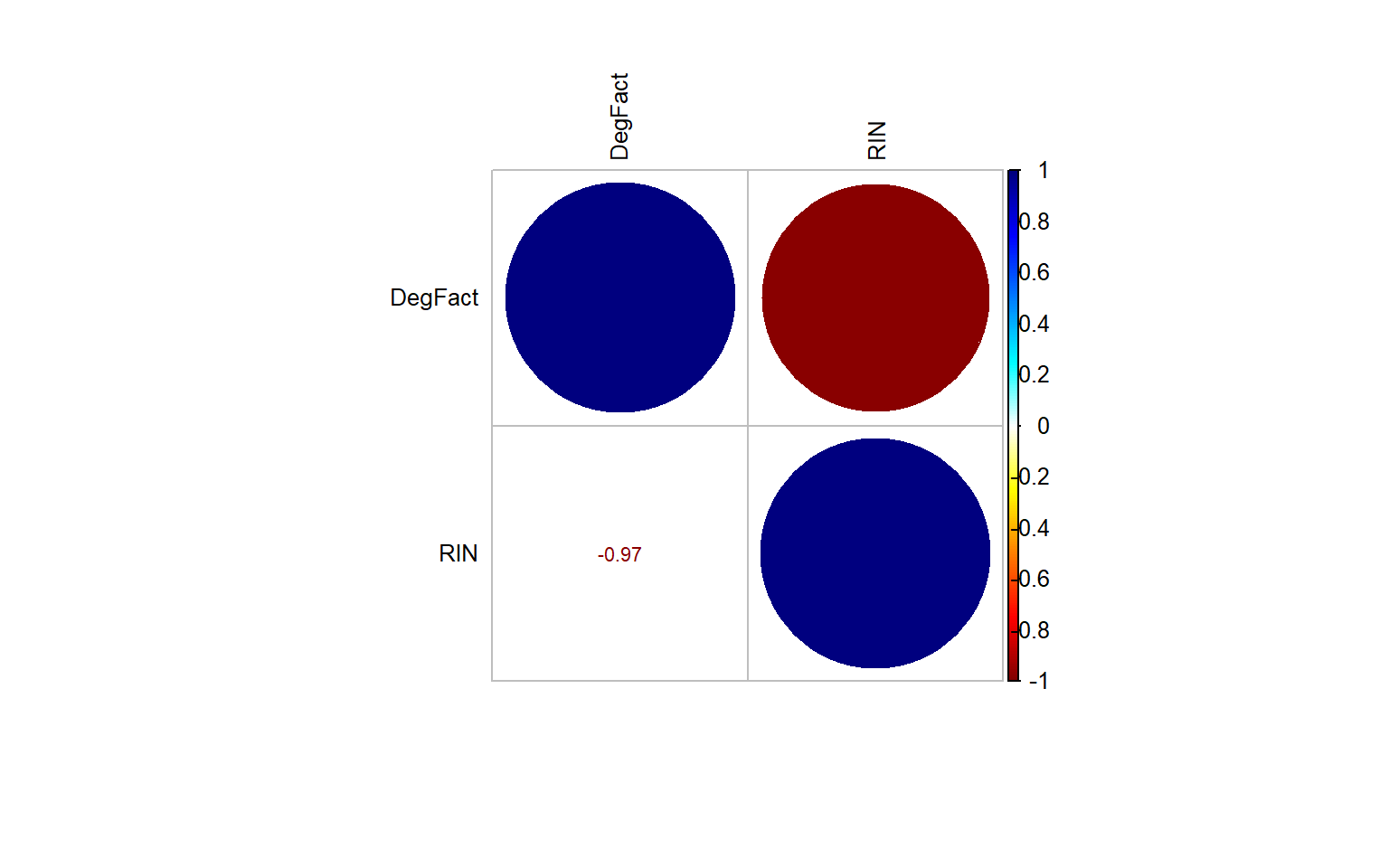# Access the correlation matrix via its first assay:
# assay(correlationSE,1)

## 3.4 Stability analysis

The calculation of the stability indices is performed by stability and stabilityRange functions.

### 3.4.1 Stability

The stability index analysis is performed by the stability function. For instance, running a stability analysis for the metrics of rnaMetrics with a number of 100 bootstrap replicates with a k-means cluster whose k is 2 (note that k must be inside [2,15] range):

stabilityData <- stability(rnaMetrics, k=2, bs = 100)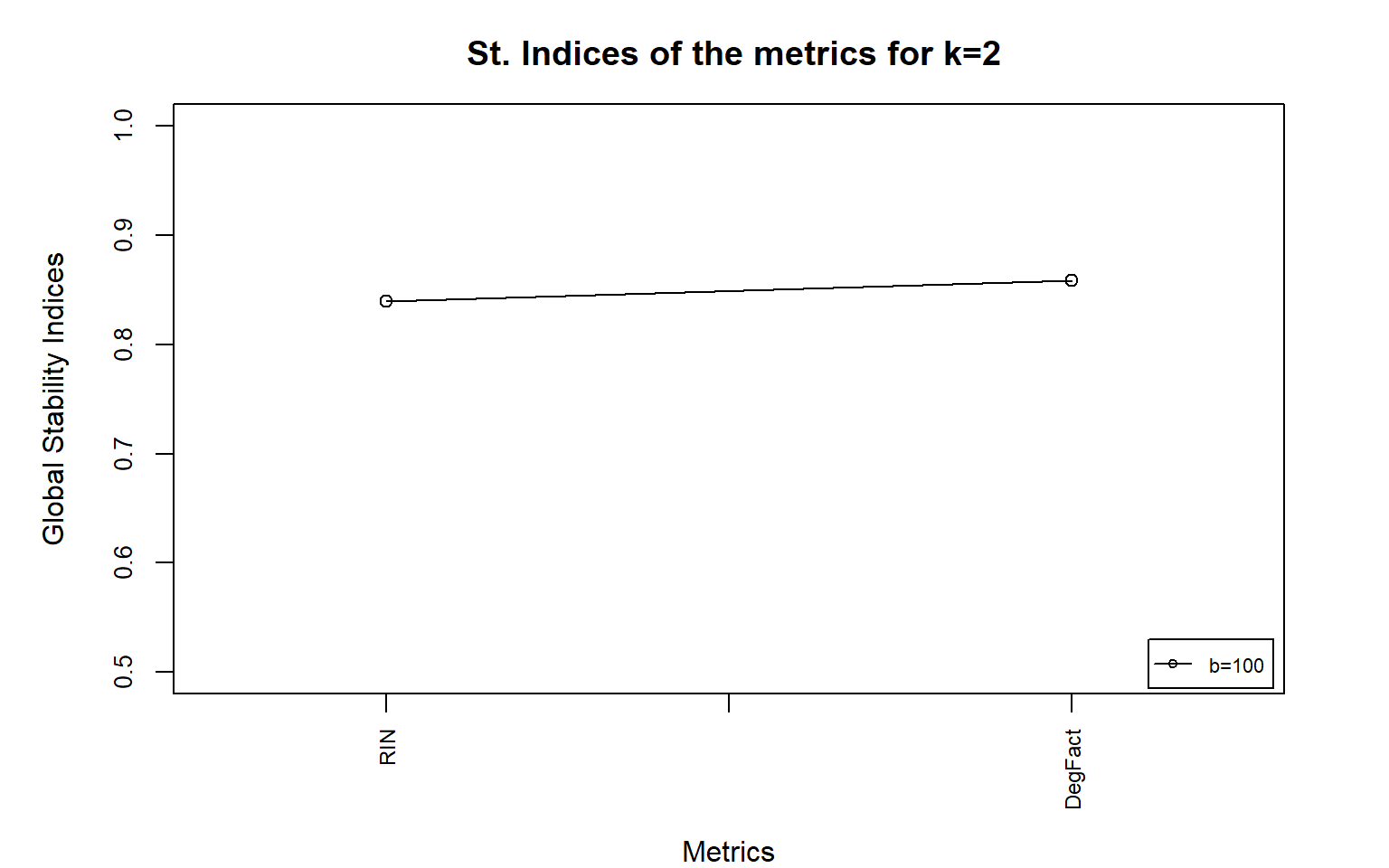The stability function returns the stabilityData object, a SummarizedExperiment that contains an assay with the information shown in the plot:

assay(stabilityData, 1)
Metric Mean_stability_k_2
RIN 0.839702380952381
DegFact 0.858073232323232

The plot represents the stability mean from each metric for a given k value. This mean is calculated by performing the average of every stability index from kranges [1,k] for each metric.

### 3.4.2 Stability range

The stabilityRange function is an iterative method of stability function. It performs a stability analysis for a range of k values (k.range).

For instance, analyzing the stability of rnaMetrics in range [2,4], with bs=100:

stabilityRangeData = stabilityRange(rnaMetrics, k.range=c(2,4), bs = 100)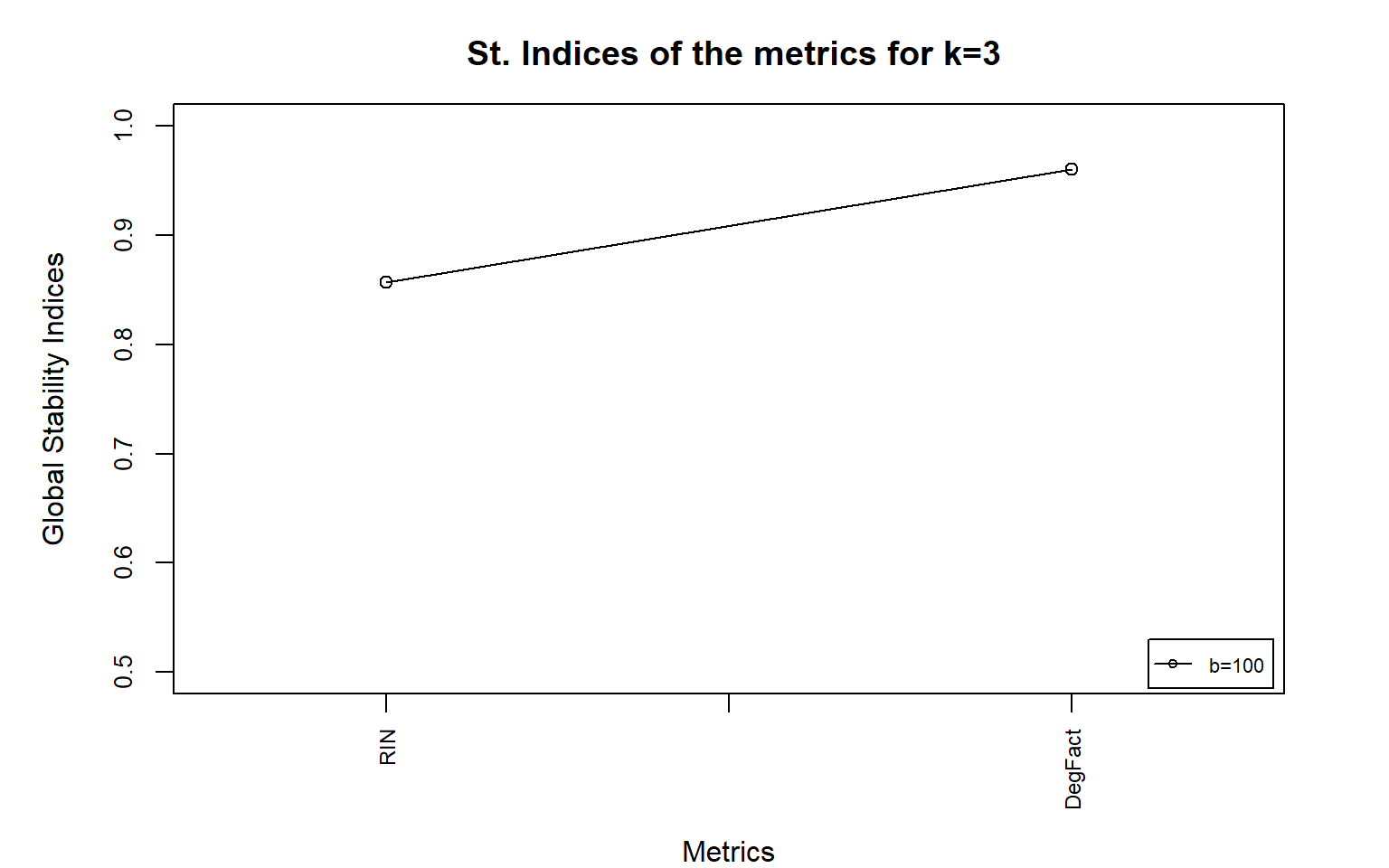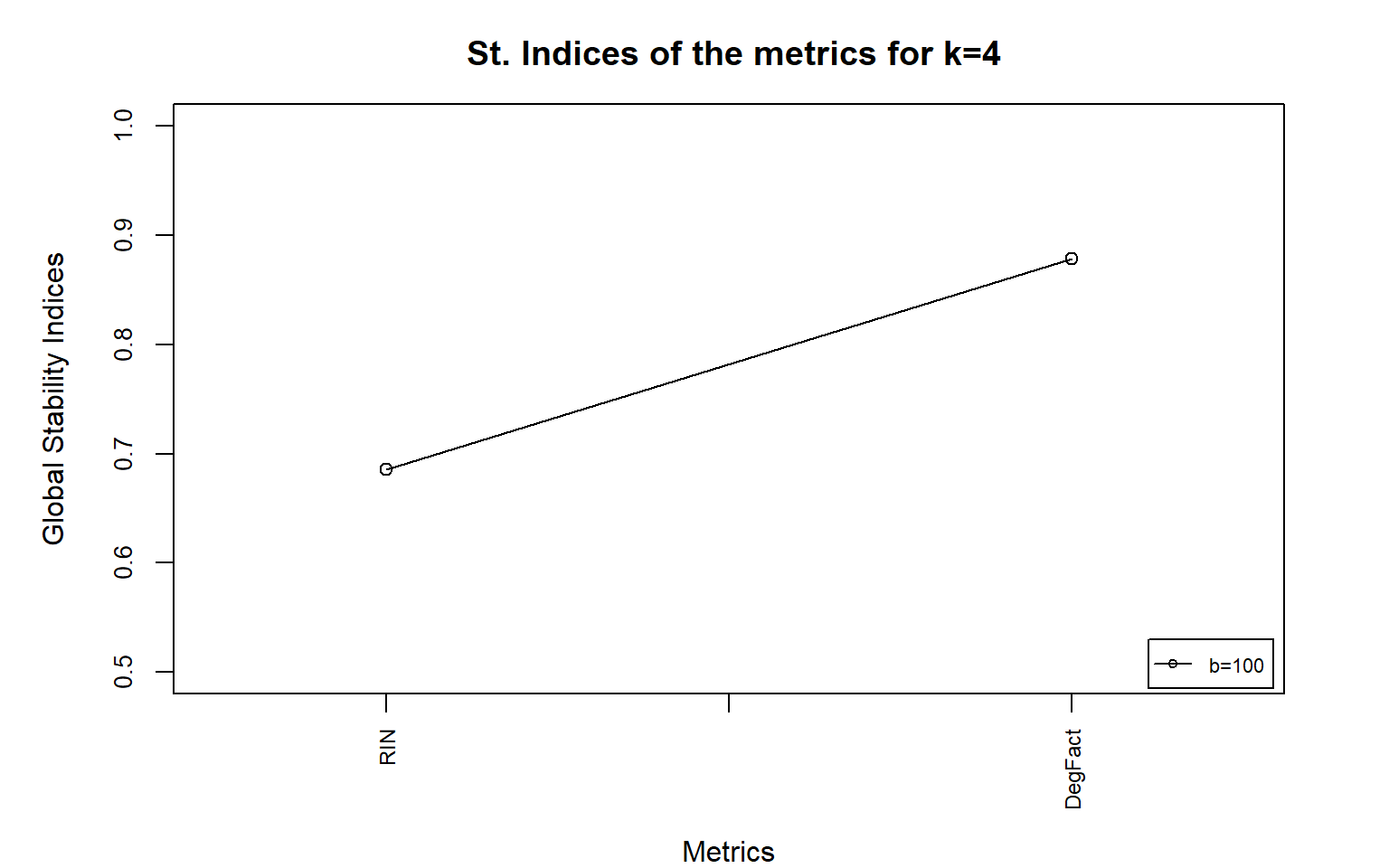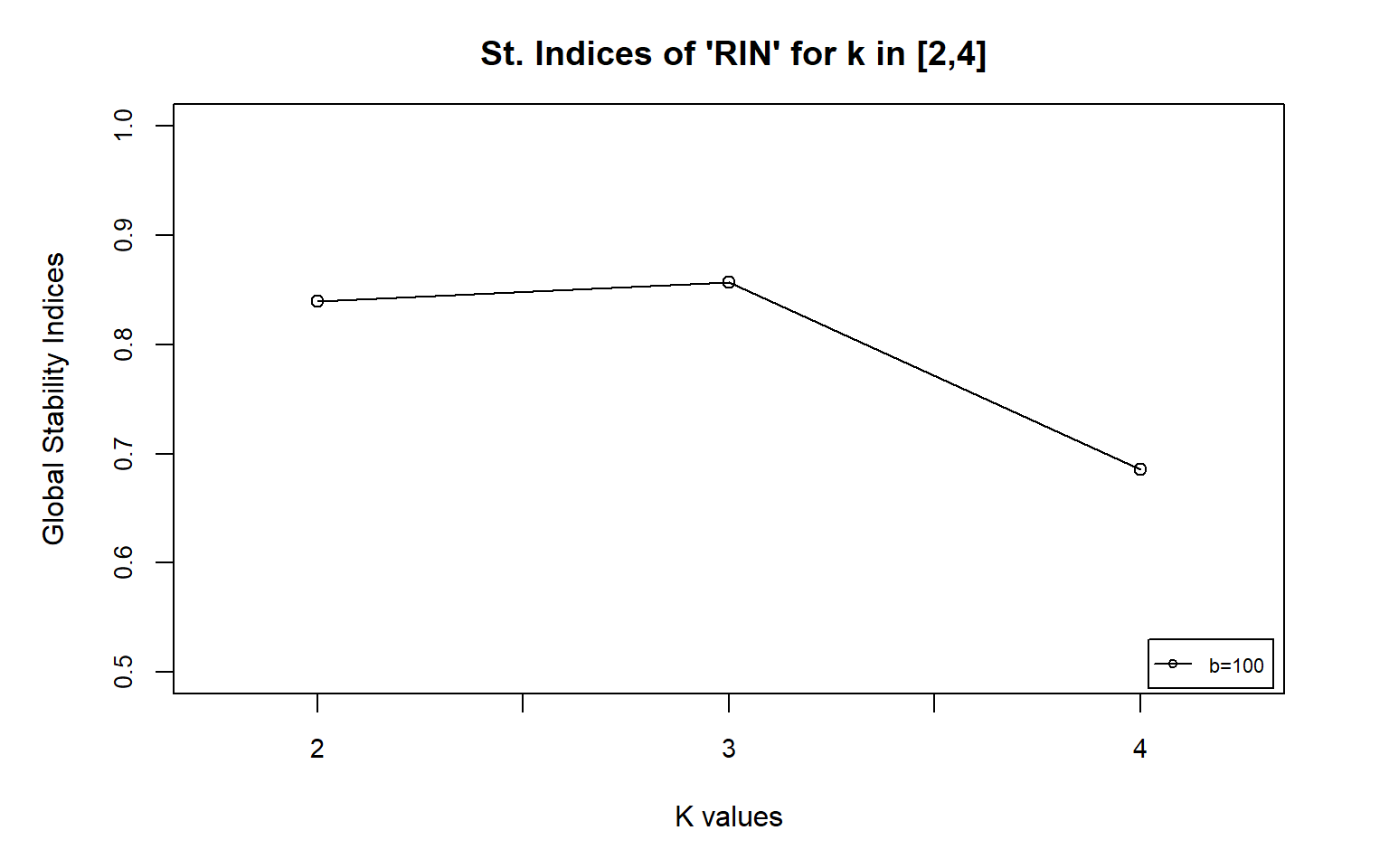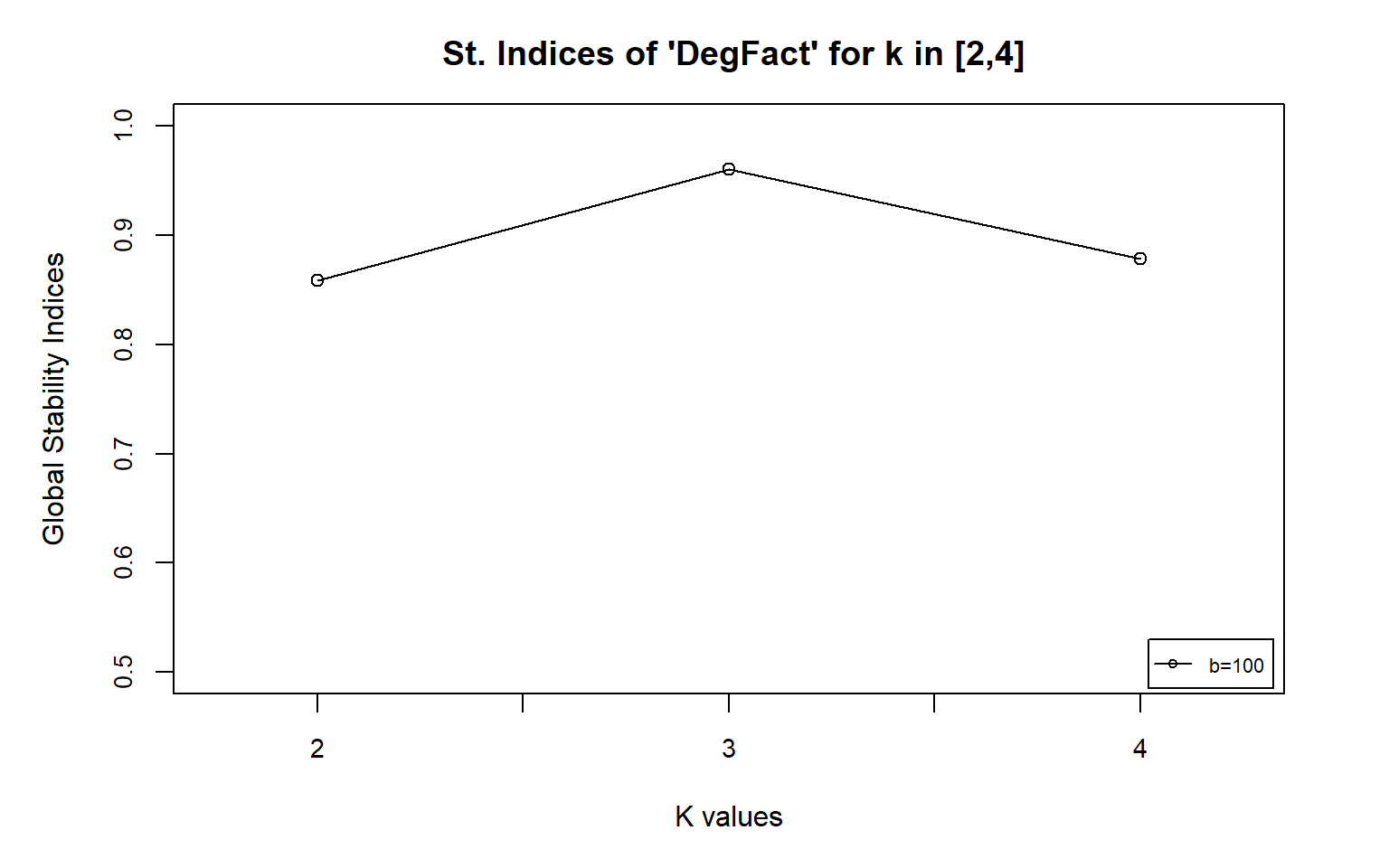Two kind of graphs are plotted in stabilityRange function. The first type (titled as “St. Indices for k=X across metrics”) shows, for every k value, the stability indices across the metrics. The second kind (titled as St. Indices for metric ‘X’ in range [x,y]), shows a plot of the behaviour of each metric across the k range.

## 3.5 Goodness of classifications

There are two methods to calculate the goodness of classifications: quality and qualityRange.

### 3.5.1 Quality

This method plots how the metrics behave for the current k value, according to the average silhouette width. Also, it will plot how the clusters are grouped for each metric (one plot per metric). For instance, running a quality analysis for the two metrics of rnaMetrics dataset, being k=4:

qualityData = quality(rnaMetrics, k = 4)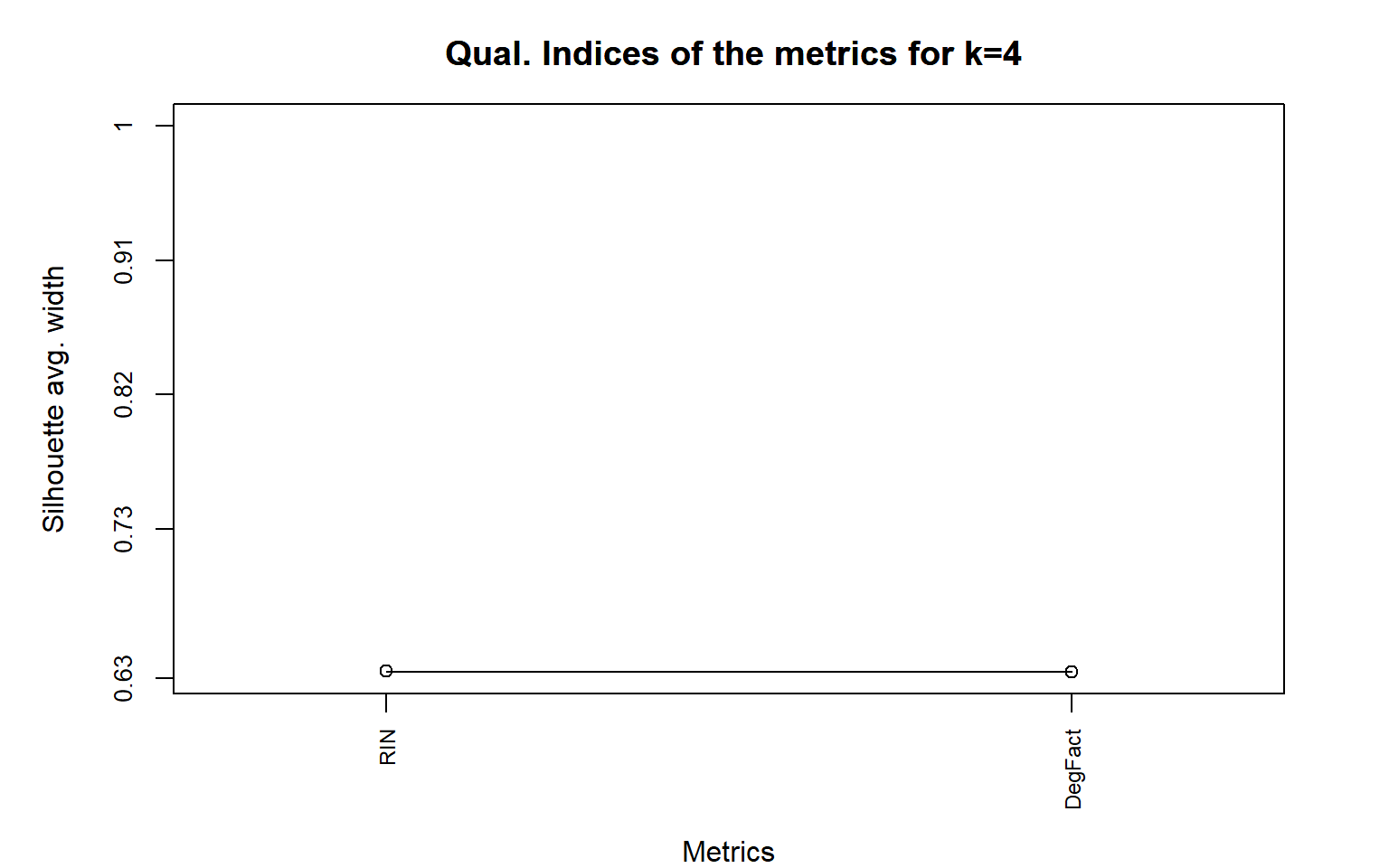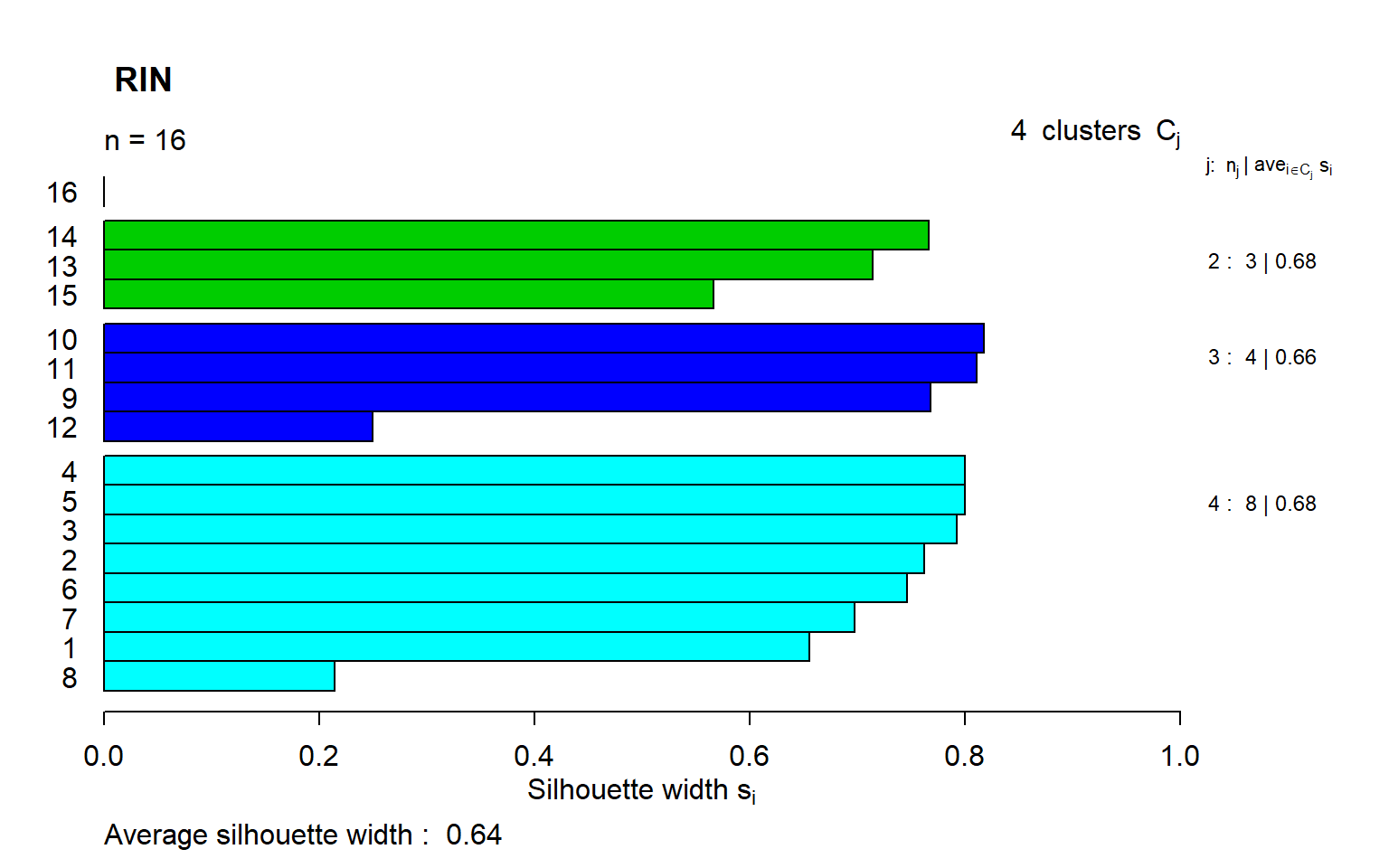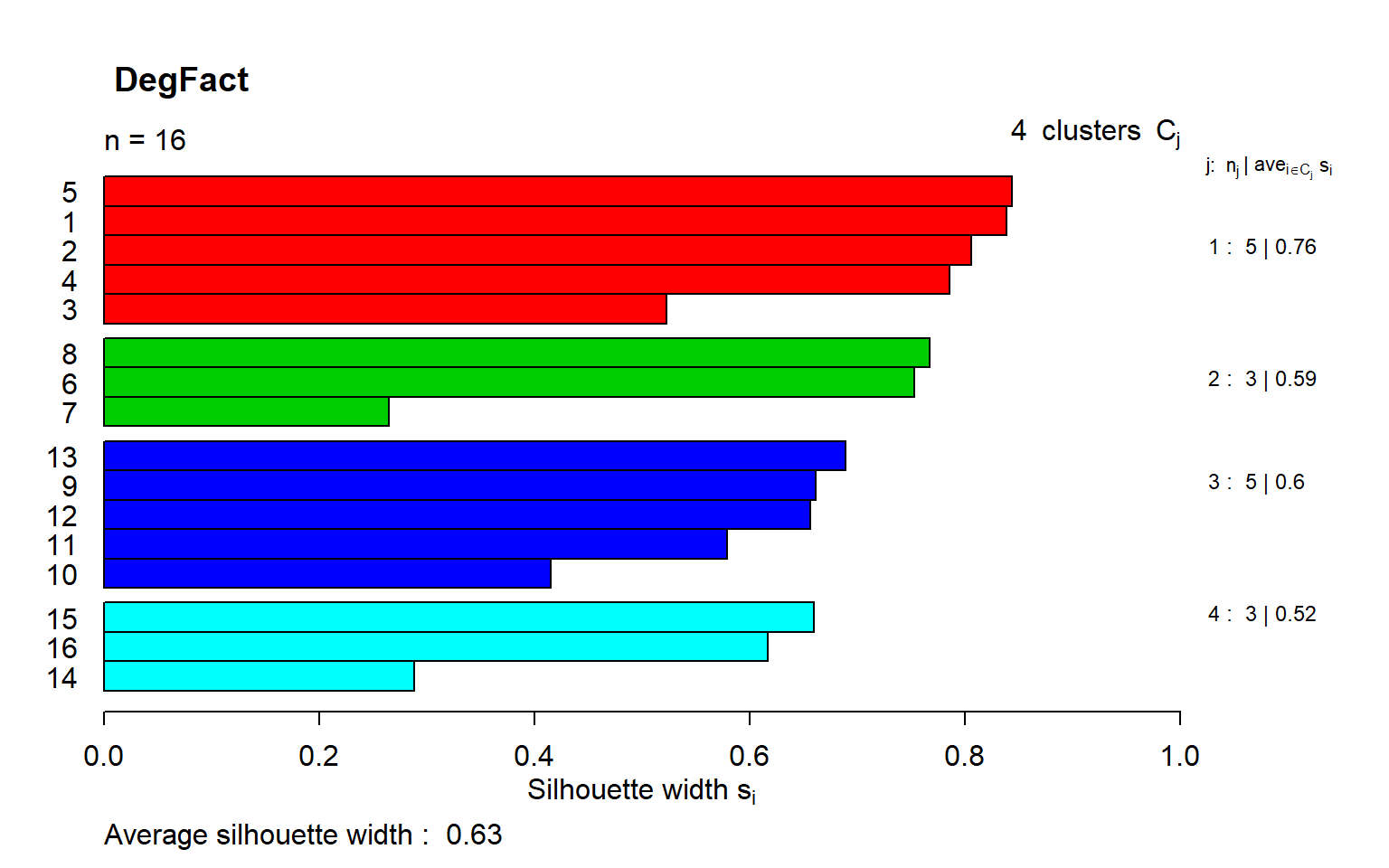The data of the first plot titled as “Qual. Indices for k=4 across metrics” according to Silhouette avg. width, is stored in Avg_Silhouette_Width column from the first assay of the SummarizedExperiment, qualityData. The other three plots titled by their metric name display the input rows grouped by colours for each cluster, along with their Silhouette width scores.

The variable qualityData contains information about the clusters of each metric: The average silhouette width per cluster, the overall average sihouette width (taking into account all the clusters) and the number of individuals per cluster:

assay(qualityData,1)
Metric Cluster_1_SilScore Cluster_2_SilScore Cluster_3_SilScore Cluster_4_SilScore Avg_Silhouette_Width Cluster_1_Size Cluster_2_Size Cluster_3_Size Cluster_4_Size
RIN 0 0.682539682539682 0.661697073729871 0.68338517747747 0.635093047647393 1 3 4 8
DegFact 0.759196481622952 0.59496499852177 0.600198799385732 0.521618857725795 0.634170498361632 5 3 5 3

### 3.5.2 Quality range

The qualityRange function is an iterative method that uses the same functionality of quality for a range of values (k.range), instead for one unique k value. This methods allows to analyse the goodness of the classifications of the metric for different values of the range.

In the next example we will keep using the rnaMetrics dataset, and a k.range set to [4,6].

k.range = c(4,6)
qualityRangeData = qualityRange(rnaMetrics, k.range)